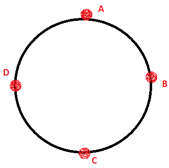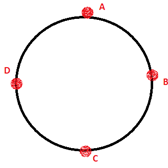Physics
Easy

Question

# A boy is sitting in a merry-go-round.  The time period of circular motion is 5 sec, and its speed of rotation is 70 m/s. What is the radius of the circle formed?

## 5.573 m55.73 m557.3 m5573 mHint:

## The correct answer is: 55.73 m

Physics

Physics

Physics

### What is the direction of the velocity at point mentioned if the ball is revolving anticlockwise?At point DPhysics

Physics

Physics

### What is the direction of the velocity at point mentioned if the ball is revolving anticlockwise?At point APhysics

Physics

Physics

### An object is moving at a uniform speed in a counterclockwise, horizontal circle. Which of the following images correctly depicts the force and velocity at a point in time?Physics

Physics

Physics

### A boy moves a tied stone above his head in circles, time period of circular motion is 2 sec and it forms a circle of 20 cm radius. What is the speed of rotation?Physics

Physics

Physics

### What is the direction of the velocity at point mentioned if the ball is revolving clockwise.At point B#### With Turito Foundation.#### Get an Expert Advice From Turito.